Egwald Mathematics: Nonlinear Dynamics:

Trygve Haavelmo Growth Model

Continuous versus Discrete Time

by

Elmer G. Wiens

Egwald's popular web pages are provided without cost to users.
Please show your support by joining Egwald Web Services as a Facebook Fan:Follow Elmer Wiens on Twitter:The Trygve Haavelmo Growth Model

The Trygve Haavelmo growth model in A Study in the Theory of Economic Evolution. provides an example of the different dynamical behaviours arising from equivalent models expressed as either differential or difference equations. While the solution trajectories of the continuous time version of the model converge to its fixed point, the solution trajectories of the discrete time version may exhibit chaotic behaviour.

As described by Hans-Walter Lorenz in Nonlinear Dynamical Economics and Chaotic Motion (141-143), the one-dimensional Haavelmo (28-29) growth cycle model describes an economy's production of output as a function of the stock of capital (K) and the level of employment (N).

Continuous Time

Using a constant returns to scale Cobb-Douglas production function version of this model:

Y = c * K(1-a) * Na,     0 < a < 1,   c > 0,         (1)

the differential equation governing the growth of employment is:

dN / dt = N * (α - β * N / Y),   N(0) = N0,     α, β > 0,         (2)

whose solution is a function N(t) = N(t, N0)) of time, t, and the initial condition, N(t=0) = N0.

Thus, the rate of growth of employment, dN/dt / N, is an increasing function of per capita income (output), Y / N.

Combining equations (1) and (2) yields:

dN / dt = f(N, α) = α * N - β / (c * K(1-a)) * N(2 - a),   N(0) = N0.         (3)

where α is the parameter of the differential equation (2) to be analyzed.

The Fixed Point

To find the fixed point, set f(N, α) = 0, and solve for N*:

f(N, α) = α * N - β / (c * K (1-a)) * N(2 - a) = 0, or

N* = (α * c * K(1-a) / β)1 / (1 - a).         (4)

Linear Stability Analysis

Evaluating the partial derivative of f with respect to N at the fixed point N* yields:

fN(N, α) = α - (2 - a) * (β / (c * K(1 - a) * N(1 - a), and

fN(N*, α) = α * (a - 1) < 0,

since α > 0 and 0 < a < 1. Thus the nonlinear process determined by the function f is stable with the fixed point at N*.

Phase Diagrams and Solution Trajectories

Particularize the continuous growth model by setting:

K = 3,     a = .3,     β = 1,     c = .9.

with parameter α and initial condition N(0) = N0.

The following diagrams show the phase diagrams and solution trajectories, N(t), for various initial conditions N(0), and two values of the parameter α.

 α = 1.5,     N* = 4.6059,     Y* = 3.0706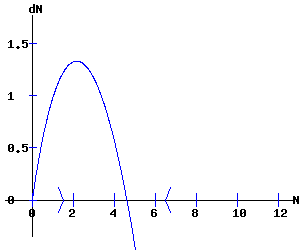α = 2.5,     N* = 9.5552,     Y* = 3.8221Discrete Time

In the differential equation (3), replace the trajectory function N(t) by the trajectory orbit {Nt}, and the differential operator dN / dt with the finite difference Nt+1 - Nt to yield the difference equation:

Nt+1 - Nt = α * Nt - β / (c * K(1-a)) * Nt(2 - a),   N0 = N0, or

Nt+1 = (1 + α) * Nt - β / (c * K(1 - a)) * Nt(2 - a),   N0 = N0.         (5)

This difference equation can be transformed by the transformation:

Nt = (K(1 - a) * (1 + α) / β)1/(1 - a) * xt

to produce the equation:

xt+1 = f(xt, α) = (1 + α) * xt * (1 - xt(1 - a)),   x0 = x0 > 0.         (6)

The dynamics of equation (6) are qualitatively equivalent to those of the logistics equation (set r = (1 + α) and let a → 0). Moreover, f(0, α) = f(1, α) = 0, and f is one-humped and noninvertible.

Fixed Points

Fixed points of the discrete f map satisfy:

f(x, α) = (1 + α) * x * (1 - x(1 - a)) = x,         (7)

which are:

x1 = 0,

x2 = [α/ (1 + α)]1/ (1 - a).         (8)

Linear Stability Analysis

The partial derivative of f with respect to x is:

fx (x, α) = (1 + α) * (1 - (2 - a)) * x (1 - a).        (9)

Evaluating f at its fixed points to obtain the multipliers:

λ1 = fx(0, α) = (1 + α), and

λ2 = fx(x2, α) = (1 + α)*(1 - (2 - a) * α/ (1 + α) = 1 - α * (1 - a).

Since α > 0 → λ1 > 1 → x1 = 0 is a repeller.   If 0 < α < 2 / (1 - a) → 1 > λ2 > -1 → x2 is an attractor.

Phase Diagrams and Solution Trajectories

Particularize the discrete growth model by setting:

a = .3.

with parameter α and initial condition x0 = x0.

The fixed point x2 of f is an attractor in the range:

0 < α < 2 / (1 - a) = 2 / .7 = 2.8571.         (10)

At α = 2 / (1 - a) = 2 / .7, the f map undergoes a flip bifurcation, where x2 switches from attractor to repeller.

The following diagrams show the phase diagrams and solution trajectory orbits, {xt}, for various initial conditions x0 and values of the parameter α.

 α = 2.5,     x1 = 0 repeller,   x2 = 0.6184 attractor.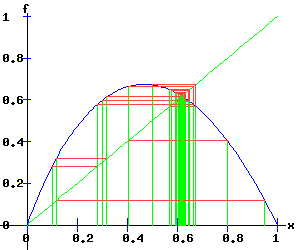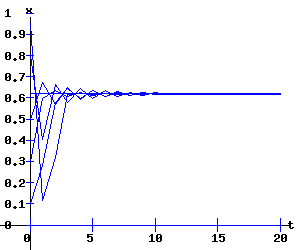α = 2 / .7 = 2.8571,     x1 = 0 repeller,   x2 = 0.651 attractor;     flip bifurcationα = 3.2,     x1 = 0 repeller,   x2 = 0.678 repeller x3 = .4659 attractor,     x4 =.81035 attractorThe Second Order Map of f, denoted by f2, is xt+2 = f(xt+1, α) = f (f(xt, α), α ) = f2(xt, α) .

The following graphs display the phase diagrams of f in blue and f2 in red. The stable fixed points x3 and x4 of f2 emerge as α increases past 2/.7. At α = 2 / .7 = 2.8571, f2 has a fixed point of multiplicity three (ie x2 = x3 = x4 = 2 / .7). For α > 2/.7, x3 and x4 bracket x2, and establish the period-2 cycle seen in the above trajectory diagram for α = 3.2. Eventually, these period-2 fixed points become unstable, and undergo flip bifurcations with respect to f4, the period doubling map of f2.

 α = 2.5 α = 2.8571     flip bifurcation α = 3.2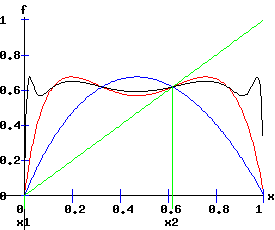Second Order (f2) Flip Bifurcation

The following diagrams display the phase diagrams and solution trajectories of x(t, x0) for the discrete time, nonlinear dynamic process. At α = 3.48365646, f2 undergoes a period doubling (flip) bifurcation with x3 and x4 switching from attractors to repellers. The trajectory xt switches between the four attracting fixed points of f4, creating a stable four-cycle.

 α = 3.4837,     x1 = 0 repeller,   x2 = 0.697 repeller; x3 = 0.4058 attractor,     x4 =0.8517 attractor;     f2flip bifurcationThe Fourth Order Map of f, denoted by f4, is xt+4 = f2 (f2(xt, α), α ) = f4(xt, α) .

The following graph displays the phase diagrams of f in blue, f2 in red, and f4 in black. The fixed points x3 and x4 of f2 flip from attractor to repeller as α increases past 3.48365646. For α > 3.48365646, four stable fixed points of f4 emerge bracketing x3 and x4, and establish the period-4 cycle. Eventually, these period-4 fixed points become unstable, and undergo flip bifurcations with respect to f8, the period doubling map of f4.

 α = 3.4837     f2 flip bifurcationFourth Order (f4) Flip Bifurcation

The following diagrams display the phase diagrams and solution trajectories of x(t, x0) for the discrete time, nonlinear dynamic process. At α = 3.61271754, f4 undergoes a period doubling (flip) bifurcation with x5, x6, x7, and x8 switching from attractors to repellers. The trajectory xt switches between the eight attracting fixed points of f8, creating a stable eight-cycle.

 α = 3.6127,     x1 = 0 repeller,   x2 = 0.705 repeller; x3 = 0.38459 repeller,     x4 = 0.86524 repeller;     x5 = 0.32709, x6 = 0.49343, x7 = 0.8187, x8 = 0.88789 attractors;   f4flip bifurcationThe following graph displays the phase diagrams of f in blue, f4 in red, and f8 in black. The fixed points x5, x6, x7, and x8 of f4 flip from attractor to repeller as α increases past 3.61271754. For α > 3.61271754, eight stable fixed points of f8 emerge bracketing x5, x6, x7, and x8, and establish the period-8 cycle. Eventually, these period-8 fixed points become unstable, and undergo flip bifurcations with respect to f16, the period doubling map of f8.

 α = 3.6127     f4 flip bifurcationPeriod Doubling Cascade

As α increases, period doublings occur as f, f2, f4, f8, . . . bifurcate at α1 = 2/.7, α2 = 3.4837, α3 = 3.6127, α4 = . . ..

Bifurcation Diagram

The intermittent emergence of order and chaos is revealed in the orbit diagram below, for α (called r) in the interval [2.8, 4].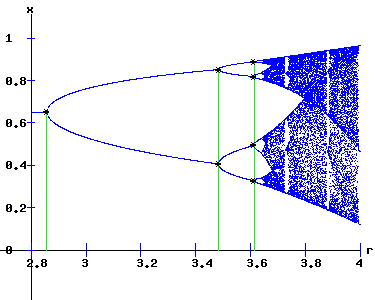The orbit:

{xt+1 = f(xt ,α), x0 = x0}

exhibits aperiodic — chaotic — behaviour, as α increases, with periodic windows appearing. These dynamics are qualitatively similar to those observed with the logistics map.

Continuous versus Discrete Time Dynamics.

The table below shows how the economy's levels of employment and output increases in a stable manner with α in the continuous time model. However, in the discrete time model, these levels of employment and output cycle and exhibit chaotic dynamics as α increases.

 Continuous Time Discrete Time α N Y x N Y 2.5 9.555 3.822 0.618 11.107 3.999 2.857 11.563 4.047 0.651 13.442 4.234 3.2 13.596 4.249 0.46590.8104 10.85918.886 3.9724.689 3.484 15.349 4.406 0.40580.8517 10.38321.793 3.9194.895 3.613 16.168 4.475 0.32710.49340.81870.8879 8.71613.14821.81523.659 3.7184.2064.8965.017

References.

• Haavelmo, Trygve. A Study in the Theory of Economic Evolution. Amsterdam: North-Holland, 1954.
• Lorenz, Hans-Walter. Nonlinear Dynamical Economics and Chaotic Motion. Berlin: Springer-Verlag, 1993.Copyright © Elmer G. Wiens:   EgwaldWeb ServicesAll Rights Reserved.    Inquiries